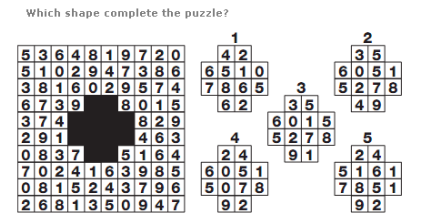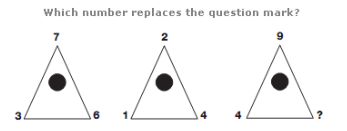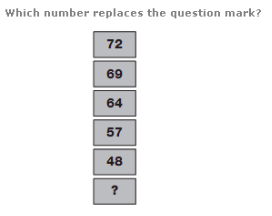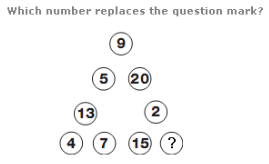61.A. 1 B. 3 C. 4 D. 5

Explanation: :

Each row of the grid contains each of the 10 digits, 0 to 9, written in a random order.

62.A. 8 B. 6 C. 14 D. 16

Explanation: :

Numbers in the segments of the lower left circle equal the sum of the numbers in the upper left and central circles.

Numbers in the segments of the lower right circle equal the sum of the numbers in corresponding segments of the upper right and central circles.

63.A. 9 B. 10 C. 13 D. 7

Explanation: :

The numbers on the points of the lower triangle equal the sum of the numbers on corresponding points of the upper two triangles.

64.A. 56 B. 35 C. 33 D. 37

Explanation: :

As you move down, subtract 3 from the previous number, then 5, 7, 9 and 11.

65.A. 13 B. 15 C. 11 D. 9